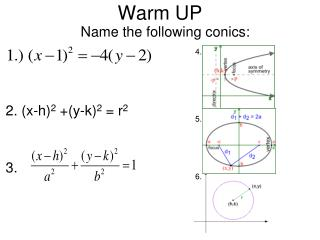Download PresentationWarm UP

Warm UP - PowerPoint PPT Presentation

4. 5. 6. Warm UP. 2. (x-h) 2 +(y-k) 2 = r 2 3. Name the following conics:. Unit 5: Non Cartesian Functions LG 5-1 Parametric Equations. Graph parametric equations. Determine an equivalent rectangular equation for parametric equations.I am the owner, or an agent authorized to act on behalf of the owner, of the copyrighted work described.
Download PresentationWarm UP

Download Policy: Content on the Website is provided to you AS IS for your information and personal use and may not be sold / licensed / shared on other websites without getting consent from its author.While downloading, if for some reason you are not able to download a presentation, the publisher may have deleted the file from their server.

- - - - - - - - - - - - - - - - - - - - - - - - - - E N D - - - - - - - - - - - - - - - - - - - - - - - - - -
Presentation Transcript
1. 4. 5. 6. Warm UP 2. (x-h)2 +(y-k)2 = r2 3. Name the following conics:

2. Unit 5: Non Cartesian FunctionsLG 5-1 Parametric Equations Graph parametric equations. Determine an equivalent rectangular equation for parametric equations. Determine parametric equations for a rectangular equation. Determine the location of a moving object at a specific time.

3. There are times when we need to describe motion (or a curve) that is not a function. We can do this by writing equations for the x and y coordinates in terms of a third variable (usually time or ). These are called parametric equations. “t” is the parameter. (It is also the independent variable)

4. The variable t (the parameter) often represents time. Picture this like a particle moving along and we know its x position over time and its y position over time. We can figure out each of these and plot them together to see the movement of the particle. Here are some examples of parametric functions

5. Graphing Parametric Equations Parametric functions represent plane curves in which x and y are functions of the third variable t.

6. Example 1 Graphing a Parametric Graph the curve represented by the equations The rectangular equation is

7. Determining a Rectangular Equation for Given Parametric Equations“Eliminating the Parameter” • Solve either equation for t. • Then substitute that value of t into the other equation. • Calculate the restrictions on the variables x and ybased on the restrictions on t.

8. Find Rectangular Equation Find a rectangular equation equivalent to

9. Graph the plane curve represented by the parametric equations t 0 1 2 3

10. Now, eliminate the parameter Solve for the parameter t in one of equations (whichever one is easier). Substitute for t in the other equation. We recognize this as a parabola opening up. Since our domain for t started at 0, it is only the right half.

11. Graph the plane curve represented by the parametric equations t 0

12. Now, eliminate the parameter. Based on our curve we'd expect to get the equation of an ellipse. 2 2 4 4 When you want to eliminate the parameter and you have trig functions, it is not easy to solve for t because it requires inverse functions. Instead you solve for cos t and sin t and substitute them in the Pythagorean Identity:

13. If we let t = the angle on the unit circle, then: Since: We could identify the parametric equations as a circle.

14. You try! Write the rectangular equation for these parametric equations This is the equation of an ellipse.